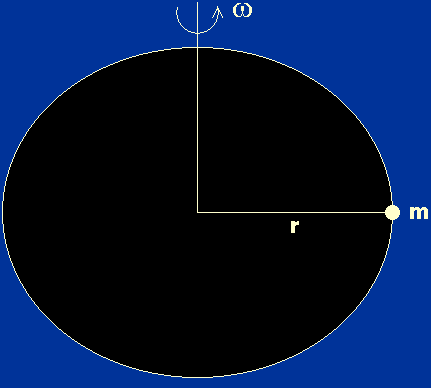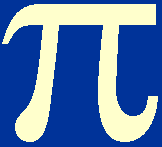Stars are rotating, gaseous bodies, so we might expect them to be flattened at the poles. But to what extent are they flattened? If the departures from spherical symmetry are negligible, then the variables in the equations of stellar structure depend only on the distance from the centre of the star. If the departures from spherical symmetry are significant, the shape of the star must be taken into account.

Consider an element of mass m near the surface of a star of mass M and radius r, as shown in figure 8.

 Figure 8: A small element of mass near the surface of a rotating star.In the absence of rotation, the inward force of gravity on the element is balanced by the outward force due to thermal pressure. With rotation, however, an additional inwardly-acting force is required to keep the element in circular motion. This centripetal force is also provided by gravitation, which means that the element must move outwards to regions of lower thermal pressure in order to maintain hydrostatic equilibrium. The centripetal force is given by

m2r,

whereis the angular velocity of the star. This force will not cause a serious departure from spherical symmetry provided that it is much less than the gravitational force acting on the element, i.e.

m2r << GMm / r2,

which on rearrangement gives<< (GM / r3)½ .

It can be seen that the right-hand side of this equation is very similar to the inverse of the expression for the dynamical timescale we obtained earlier,

td = (2r3 / GM)½.

In fact, given that= 2/ P, where P is the rotation period of the star, we can say that rotation will have only a slight influence on the structure of a star provided that the rotation period is very long compared to the dynamical timescale of the star.

The Sun rotates once in just under a month. The dynamical timescale of the Sun is around 2000 s. Hence, we may conclude that:

departures from spherical symmetry due to rotation can be neglected.

This statement is true for the vast majority of stars. There are some stars which rotate much more rapidly than the Sun, however, and for these the rotation-distorted shape of the star must be accounted for in the equations of stellar structure.